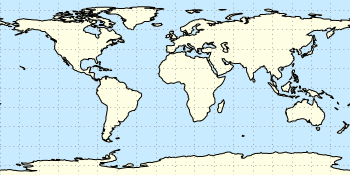# Gnuplotting

Create scientific plots using gnuplot

April 3rd, 2013 | 3 Comments

In one of the last posts, we came up with an updated data set representing the world. One way to plot this data set is with a 2D plot, as shown in Fig. 2. But if you compare the output with the one you see for example at Google Maps you will noticed a difference. That is due to the fact that Google uses the Mercator projection of the data. This projection preserves the angles around any point on the map, what is useful if you have a close look at some streets. The disadvantage of the Mercator projection is the inaccuracy of the sizes of the countries near to the poles. For example the size of Greenland is completely overemphasized as you can see in Fig. 1.Fig. 1 Mercator projection of the world (code to produce this figure, data)

In order to achieve the Mercator projection, we apply the following function.

```set angles degrees
mercator(latitude) = log( tan(180/4.0 + latitude/2.0) )
set yrange [-3.1:3.1]
plot 'world_110m.txt' u 1:(mercator(\$2)) w filledcu ls 2
```Fig. 2 Equirectangular projection of the world (code to produce this figure, data)

By just plotting the data as we have done for Fig. 2, we have the Equirectangular projection with constant spacing between the latitudes and meridians. The blue background color in the first two figures can be achieved directly with a terminal setting.

```set terminal pngcairo size background '#c8ebff'
```Fig. 3 Mapping of the Mercator projection (code to produce this figure)

In Fig. 3 the Mercator projection function is shown as an input-output-function of the latitude values. The placing of the latitude values on the y-axis can be easily done with a loop.

```set ytics 0
do for [angle=-80:80:20] {
}
```

1.Thor says:

This is great stuff :-), thanks for sharing.

2.Jose Gama says:

Here is a library of gnuplot functions for cartographic projections in 2D and 3D

https://github.com/tuxcell/gnuplotcartography
run
gnuplot demo1.gnu
gnuplot demo2.gnu
gnuplot demo3.gnu

And an R-package (Programming interface):
CRAN – Package Rgnuplot
https://cran.r-project.org/web/packages/Rgnuplot/index.html

The R package can create maps for gnuplot from image files (jpeg, PNG, etc) or shapefiles and plot them in over a dozen cartographic projections

3.hagen says:

Very nice, thanks!